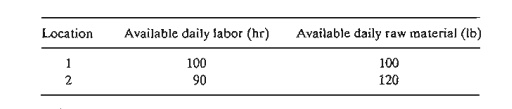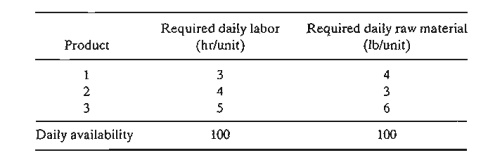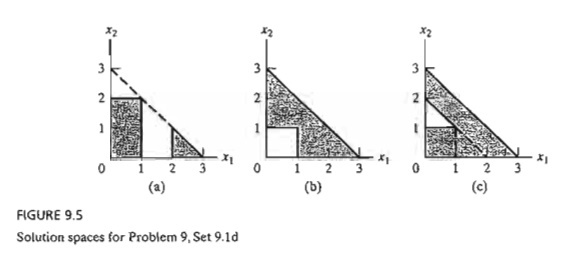Home | | Resource Management Techniques | Either Or and If Then Constraints- Integer Linear Programming Illustrative Applications

# Either Or and If Then Constraints- Integer Linear Programming Illustrative Applications

In this section, we deal with models in which constraints are not satisfied simultaneously (either-or) or are dependent (if-then), again using binary variables.

Either-Or and If-Then Constraints

In the fIxed-charge problem (Section 9.1.3), we used binary variables to handle the discontinuity in the objective cost function. In this section, we deal with models in which constraints are not satisfied simultaneously (either-or) or are dependent (if-then), again using binary variables. The transformation does not change the "or" or "dependence" nature of the constraints. It simply uses a mathematical trick to present them in the desired format of "and" constraints.

Example 9.1-4   (Job-Sequencing Model)

Jobco uses a single machine to process three jobs. Both the processing time and the due date (in days) for each job are given in the following table. The due dates are measured from zero, the assumed start time of the first job.The objective of the problem is to determine the minimum late-penalty sequence for processing the three jobs.

DefineThe problem has two types of constraints: the noninterference constraints (guaranteeing that no two jobs are processed concurrently) and the due-da te constraints. Consider the noninterference constraints first.

Two jobs i and j with processing time pi and pj will not be processed concurrently if either xi xj + pj or xj xi + pi, depending on whether job j precedes job i, or vice versa. Because all mathematical programs deal with simultaneous constraints only, we transform the either-or constraints by introducing the following auxiliary binary variable:For M sufficiently large, the either-or constraint is converted to the following two simultaneous constraintsThe conversion guarantees that only one of the two constraints can be active at anyone time. If yij = 0, the first constraint is active, and the second is redundant (because its left-hand side will include M, which is much larger than pi). If yij = 1, the first constraint is redundant, and the second is active.

Next, the due-date constraint is considered. Given that dj is the due date for job j, let sj be an unrestricted variable. Then, the associated constraint isThe integer variables, y12, y13, and y23, are introduced to convert the either-or constraints into simultaneous constraints. The resulting model is a mixed ILP.

To solve the model, we choose M = 100, a value that is larger than the sum of the processing times for all three activities.

The optimal solution is xl = 20, x2, =0, and x3 = 25, This means that job 2 starts at time 0, job 1 starts at time 20, and job 3 starts at time 25, thus yielding the optimal processing sequence 2 -> 1 -> 3. The solution calls for completing job 2 at time 0 + 20 = 20, job 1 at time = 20 + 5 = 25, and job 3 at 25 + 15 = 40 days. Job 3 is delayed by 40 - 35 = 5 days past its due date at a cost of 5 X \$34 = \$170.

AMPl Moment

File ampIEx9.1-4.txt provides the AMPL model for the problem of Example 9.1-4. The model is self-explanatory because it is a direct translation of the general mathematical model given above. It can handle any number of jobs by changing the input data. Note that the model is a direct function of the raw data: processing time p, due date d, and delay penalty perDayPenal ty.Example 9.1-5    (Job Sequencing Model Revisited)

In Example 9.1-4, suppose that we have the following additional condition: If job i precedes job j then job k must precede job m. Mathematically, this if-then condition is translated asGiven ε > 0 and infinitesimally small and M sufficiently large, this condition is equivalent to the following two simultaneous constraints:If xi + pixj, then xj - (xi + pi)0, which requires w = 0, and the second constraint be-comes xk + pk  ≤  x m , as desired. Else, w  may assume the value 0 or 1, in which case the second constraint mayor may not be satisfied, depending on other conditions in the model

PROBLEM SET 9.10

*1. A game board consists of nine equal squares. You are required to fill each square with a number between 1 and 9 such that the sum of the numbers in each row, each column, and each diagonal equals 15. Additionally, the numbers in all the squares must be distinct. Use ILP to determine the assignment of numbers to squares.

2. A machine is used to produce two interchangeable products. The daily capacity of the machine can produce at most 20 units of product 1 and 10 units of product 2. Alterna-tively, the machine can be adjusted to produce at most 12 units of product 1 and 25 units of product 2 daily. Market analysis shows that the maximum daily demand for the two products combined is 35 units. Given that the unit profits for the two respective products are \$10 and \$12, which of the two machine settings should be selected? For-mulate the problem as an ILP and find the optimum. [Note: This two-dimensional prob-lem can be solved by inspecting the graphical solution space. This is not the case for the n-dimensional problem.]

*3. Gapco manufactures three products, whose daily labor and raw material requirements are given in the following table.The profits per unit of the three products are \$25, \$30, and \$22, respectively. Gapco has two options for locating its plant. The two locations differ primarily in the availability of labor and raw material, as shown in the following table:Formulate the problem as an ILP, and determine the optimum location of the plant.

4. Jobco Shop has 10 outstanding jobs to be processed on a single machine. The following table provides processing times and due dates. All times are in days and due time is measured from time 0:If job 4 precedes job 3, then job 9 must precede job 7. The objective is to process a1l1O jobs in the shortest possible time. Formulate the model as an ILP and determine the opti-mum solution by modifying AMPL file ampIEx9.1-4.txt.

5. In Problem 4, suppose that job 4 cannot be processed until job 3 has been completed.

Also, machine settings for jobs 7 and 8 necessitate processing them one right after the other (Le., job 7 immediately succeeds or immediately precedes 8). lobco's objective is to process all ten jobs with the smal1est sum of due-time violations. Formulate the model mathematically and determine the optimum solution.

6.Jaco owns a plant in which three products are manufactured. The labor and raw material requirements for the three products are given in the following table.The profits per unit for the three products are \$25, \$30, and \$45, respectively. If product 3 is to be manufactured at all, then its production level must be at least 5 units daily. For-mulate the problem as a mixed ILP, and find the optimal mix.7. UPak is a subsidiary of an LTL (Iess-than-truck-load) transportation company. Cus-tomers bring their shipments to the UPak terminal to be loaded on the trailer and can rent space up to 36 ft. The customer pays for the exact linear space (in foot increments) the shipment occupies. No partial shipment is allowed, in the sense that the entire ship-ment per customer must be on the same trailer. A movable barrier, called bulkhead, is in-stalled to separate different shipments. The per-foot fee UPak collects depends on the destination of the shipment: The longer the trip, the higher the fee. The following table provides the outstanding orders UPak needs to process.The terminal currently has two trailers ready to be loaded. Detennine the priority orders that will maximize the total income from the two trailers. (Hint A formulation using binary xij to represent load i on trailer j is straightforward. However, you are challenged to define Xij as feet assigned to load i in trailer j. The use if-then constraint to prevent partial load shipping.)

8. Show how the nonconvex shaded solution spaces in Figure 9.5 can be represented by a set of simultaneous constraints. Find the optimum solution that maximizes z = 2x\ + 3X2 subject to the solution space given in (a).

9. Suppose that it is required that any k out of the following m constraints must be active:Show how this condition may be represented.

10. In the following constraint, the right-hand side may assume one of values, bI, b2 , .. , and bmStudy Material, Lecturing Notes, Assignment, Reference, Wiki description explanation, brief detail
Operations Research: An Introduction : Integer Linear Programming : Either Or and If Then Constraints- Integer Linear Programming Illustrative Applications |